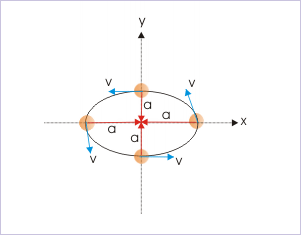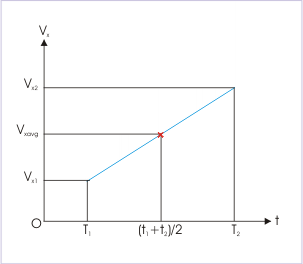# 2.4 Constant acceleration  (Page 3/4)

 Page 3 / 4The object moves along a circular path constant speed.

Notwithstanding the constant magnitude, acceleration of uniform circular motion is a variable acceleration in the horizontal plane, because direction of radial centripetal acceleration (shown with red arrow) keeps changing with time. Therefore, the acceleration of the motion keeps changing and is not independent of time as required for acceleration to be constant.

## Equation of motion

The motion under constant acceleration allows us to describe accelerated motion, using simple mathematical construct. Here, we set out to arrive at these relationships in the form of equations. In these equations, “ u ” stands for initial velocity, “ v ” for final velocity, “ a ” for constant acceleration and “t” for time interval of motion under consideration. This is short of convention followed by many text books and hence the convention has been retained here.

## First equation

$\begin{array}{ll}\mathrm{1:}& \mathbf{v}=\mathbf{u}+\mathbf{a}t\end{array}$

This relation can be established in many ways. One of the fundamental ways is to think that velocity is changed by constant acceleration (vector) at the end of successive seconds. Following this logic, the velocity at the end of t successive seconds are as under :

$\begin{array}{lll}\text{At the end of}\phantom{\rule{2pt}{0ex}}{1}^{\mathrm{st}}\phantom{\rule{2pt}{0ex}}\text{second}& :& \mathbf{u}+\mathbf{a}\\ \text{At the end of}\phantom{\rule{2pt}{0ex}}{2}^{\mathrm{nd}}\phantom{\rule{2pt}{0ex}}\text{second}& :& \mathbf{u}+2\mathbf{a}\\ \text{At the end of}\phantom{\rule{2pt}{0ex}}{3}^{\mathrm{rd}}\phantom{\rule{2pt}{0ex}}\text{second}& :& \mathbf{u}+3\mathbf{a}\\ \text{At the end of}\phantom{\rule{2pt}{0ex}}{4}^{\mathrm{th}}\phantom{\rule{2pt}{0ex}}\text{second}& :& \mathbf{u}+4\mathbf{a}\\ \text{-------------------------------}\\ \text{-------------------------------}\\ \text{At the end of}\phantom{\rule{2pt}{0ex}}{t}^{\mathrm{th}}\phantom{\rule{2pt}{0ex}}\text{second}& :& \mathbf{u}+t\mathbf{a}=\mathbf{u}+\mathbf{a}t\end{array}$

We can also derive this equation, using the defining concept of constant acceleration. We know that :

$\begin{array}{l}\mathbf{a}={\mathbf{a}}_{\mathrm{avg}}\\ ⇒\mathbf{a}=\frac{\Delta \mathbf{v}}{\Delta t}=\frac{\mathbf{v}-\mathbf{u}}{t}\\ ⇒\mathbf{a}t=\mathbf{v}-\mathbf{u}\\ ⇒\mathbf{v}=\mathbf{u}+\mathbf{a}t\end{array}$

Alternatively (using calculus), we know that :

$\begin{array}{l}\mathbf{a}=\frac{đ\mathbf{v}}{đt}\\ ⇒đ\mathbf{v}=\mathbf{a}đt\end{array}$

Integrating on both sides, we have :

$\begin{array}{l}\Delta \mathbf{v}=\mathbf{a}\Delta t\\ ⇒{\mathbf{v}}_{2}-{\mathbf{v}}_{1}=\mathbf{a}t\\ ⇒\mathbf{v}-\mathbf{u}=\mathbf{a}t\\ ⇒\mathbf{v}=\mathbf{u}+\mathbf{a}t\end{array}$

## Second equation

$\begin{array}{ll}\mathrm{2:}& {\mathbf{v}}_{\mathrm{avg}}=\frac{\mathbf{u}+\mathbf{v}}{2}\end{array}$

The equation $\mathbf{v}=\mathbf{u}+\mathbf{a}t$ is a linear relationship. Hence, average velocity is arithmatic mean of initial and final velocities :Average velocity is equal to mean velocity.

$\begin{array}{l}⇒{\mathbf{v}}_{\mathrm{avg}}=\frac{\mathbf{u}+\mathbf{v}}{2}\end{array}$

## Third equation

$\begin{array}{ll}\mathrm{3:}& \mathbf{s}=\Delta \mathbf{r}={\mathbf{r}}_{2}-{\mathbf{r}}_{1}=\mathbf{u}t+\frac{1}{2}\mathbf{a}{t}^{2}\end{array}$

This equation is derived by combining the two expressions available for the average velocity.

$\begin{array}{l}{\mathbf{v}}_{\mathrm{avg}}=\frac{\Delta \mathbf{r}}{\Delta t}=\frac{{\mathbf{r}}_{2}-{\mathbf{r}}_{1}}{t}\end{array}$

and

$\begin{array}{l}{\mathbf{v}}_{\mathrm{avg}}=\frac{\left({\mathbf{v}}_{1}+{\mathbf{v}}_{2}\right)}{2}=\frac{\left(\mathbf{v}+\mathbf{u}\right)}{2}\end{array}$

Combining two expressions of average acceleration and rearranging, we have :

$\begin{array}{l}⇒\left({\mathbf{r}}_{2}-{\mathbf{r}}_{1}\right)=\frac{\left(\mathbf{v}+\mathbf{u}\right)t}{2}\end{array}$

Using the relation, $\mathbf{v}=\mathbf{u}+\mathbf{a}t$ and substituting for v, we have :

$\begin{array}{l}⇒\left({\mathbf{r}}_{2}-{\mathbf{r}}_{1}\right)=\frac{\left(\mathbf{u}+\mathbf{a}t+\mathbf{u}\right)t}{2}\\ ⇒\mathbf{s}=\Delta \mathbf{r}={\mathbf{r}}_{2}-{\mathbf{r}}_{1}==\mathbf{u}t+\frac{1}{2}\mathbf{a}{t}^{2}\end{array}$

Alternatively, we can derive this equation using calculus. Here,

$\begin{array}{l}\mathbf{v}=\frac{đ\mathbf{r}}{đt}\\ ⇒đ\mathbf{r}=\mathbf{v}đt\end{array}$

Integrating between the limits on both sides,

$\begin{array}{l}\int đ\mathbf{r}=\int \mathbf{v}đt\end{array}$

Now substituting v ,

$\begin{array}{l}⇒\int đ\mathbf{r}=\int \left(\mathbf{u}+\mathbf{a}t\right)đt\\ ⇒\Delta \mathbf{r}=\int \mathbf{u}t+\int \mathbf{a}tđt\\ ⇒\mathbf{s}=\Delta \mathbf{r}={\mathbf{r}}_{2}-{\mathbf{r}}_{1}=\mathbf{u}t+\frac{1}{2}\mathbf{a}{t}^{2}\end{array}$

The expression for the displacement has two terms : one varies linearly ( u t) with the time and the other ( $\frac{1}{2}\mathbf{a}{t}^{2}$ ) varies with the square of time. The first term is equal to the displacement due to non-accelerated motion i.e the displacement when the particle moves with uniform velocity, u . The second term represents the contribution of the acceleration (change in velocity) towards displacement.

This equation is used for determining either displacement(Δ r ) or position( ${\mathbf{r}}_{1}$ ). A common simplification, used widely, is to consider beginning of motion as the origin of coordinate system so that

#### Questions & Answers

What are the system of units
Jonah Reply
A stone propelled from a catapult with a speed of 50ms-1 attains a height of 100m. Calculate the time of flight, calculate the angle of projection, calculate the range attained
Samson Reply
58asagravitasnal firce
Amar
water boil at 100 and why
isaac Reply
what is upper limit of speed
Riya Reply
what temperature is 0 k
Riya
0k is the lower limit of the themordynamic scale which is equalt to -273 In celcius scale
Mustapha
How MKS system is the subset of SI system?
Clash Reply
which colour has the shortest wavelength in the white light spectrum
Mustapha Reply
how do we add
Jennifer Reply
if x=a-b, a=5.8cm b=3.22 cm find percentage error in x
Abhyanshu Reply
x=5.8-3.22 x=2.58
sajjad
what is the definition of resolution of forces
Atinuke Reply
what is energy?
James Reply
Ability of doing work is called energy energy neither be create nor destryoed but change in one form to an other form
Abdul
motion
Mustapha
highlights of atomic physics
Benjamin
can anyone tell who founded equations of motion !?
Ztechy Reply
n=a+b/T² find the linear express
Donsmart Reply
أوك
عباس
Quiklyyy
Sultan Reply
Moment of inertia of a bar in terms of perpendicular axis theorem
Sultan Reply
How should i know when to add/subtract the velocities and when to use the Pythagoras theorem?
Yara Reply

### Read also:

#### Get the best Physics for k-12 course in your pocket!

Source:  OpenStax, Physics for k-12. OpenStax CNX. Sep 07, 2009 Download for free at http://cnx.org/content/col10322/1.175
Google Play and the Google Play logo are trademarks of Google Inc.

Notification Switch

Would you like to follow the 'Physics for k-12' conversation and receive update notifications?ByBy